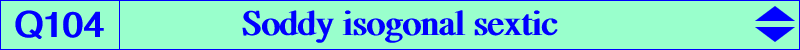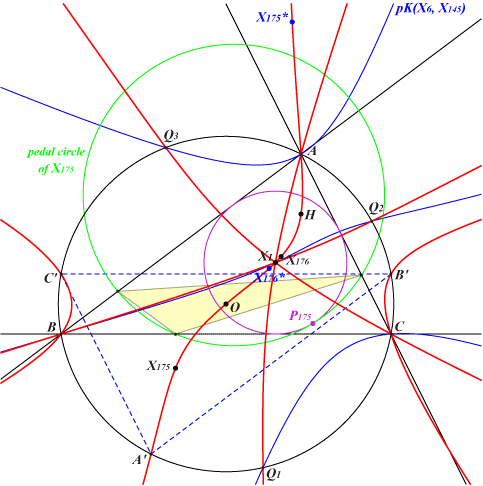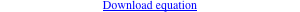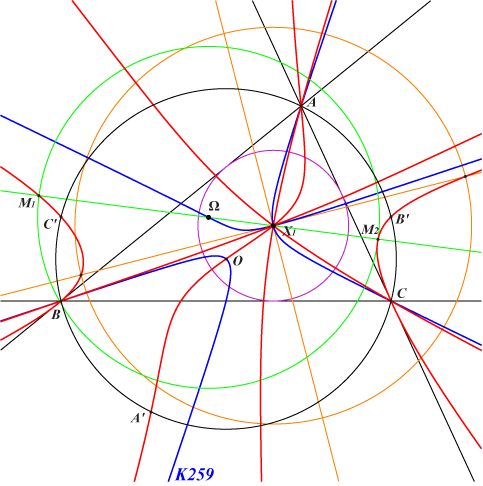too complicated to be written here. Click on the link to download a text file.X(1), X(3), X(4), X(175), X(176), X(18283), X(30336), X(30335), X(46370) X(30336), X(30335) are the isogonal conjugates of X(175), X(176) isogonal conjugates of X(18283), X(46370) reflections A', B', C' of A, B, C about O infinite points of the altitudes Q1, Q2, Q3 : on (O) and K1086 = pK(X6, X145) their isogonal conjugates at infinity vertices of the cevian triangles of X(175), X(176)Q104 is the locus of P whose pedal circle (C) is tangent to the incircle (I). This answers a question raised by Tran Quang Hung, ADGEOM #1632. This same question had been actually already asked by John Conway and solved by Jean-Pierre Ehrmann in May, 2001. See Hyacinthos #2902-2907. Recall that the locus of P whose pedal circle (C) is tangent to the nine point circle is the McCay cubic K003. *** Q104 is an isogonal sextic since two isogonal conjugate points share the same pedal circle. The incenter X(1) is a quadruple point and A, B, C are double points with tangents passing through X(30335), X(30336). Let T be X(188) or one vertex of its anticevian triangle. Let T' be the barycentric product T x X(1) and T" the T'-Ceva conjugate of X(1). The line passing through X(1) and T" is one tangent at X(1) to Q104. With T = X(188), we find T" = X(503). Q104 has three concurring asymptotes which are the parallels at X(1) to the altitudes of ABC and three other asymptotes which are parallel to those of K1086 = pK(X6, X145). Q104 meets the circumcircle at 12 points namely : – A, B, C counting for six, – A', B', C' whose Simson lines are the sidelines of ABC, – Q1, Q2, Q3 whose Simson lines are also tangent to the incircle. Their reflections about X(1) are actually the sidelines of triangle Q1Q2Q3. Note that the tangents at these three points to Q104 concur at X(55). In other words, Q1Q2Q3 is a poristic triangle. Naturally, with P = O and P* = H we find the nine point circle tangent at X(11) to (I). With P = X(175) and P = X(176) we find two remarkable circles. The contacts with (I) are now X(22106), X(22107) in ETC (2018-09-04).Any line passing through X(1) meets Q104 at two other points M1, M2 whose midpoint Ω lies on the cubic K259. Q104 and K259 have 18 common points namely : • A, B, C counting for 6, • X(1) counting for 8, • X(3) and three points at infinity, those of pK(X6, X145). The line passing through X(3) is the tangent at X(3) to Q104 in which case M1 = M2 = X(3). The Soddy line X(1)X(7) meets Q104 at the Soddy points X(175), X(176) and K259 at their midpoint X(3160). *** Q174 is another isogonal sextic with a very similar shape.